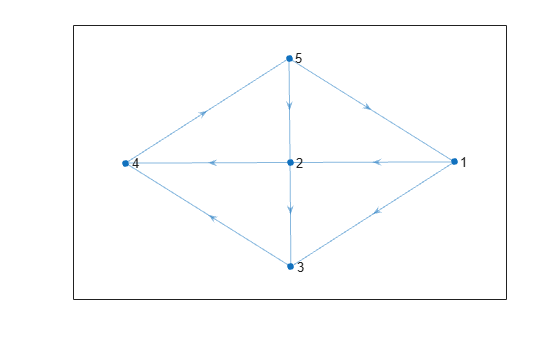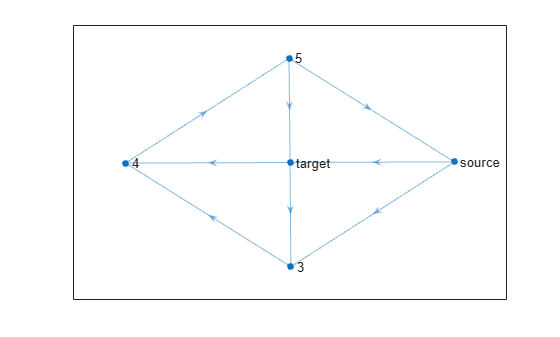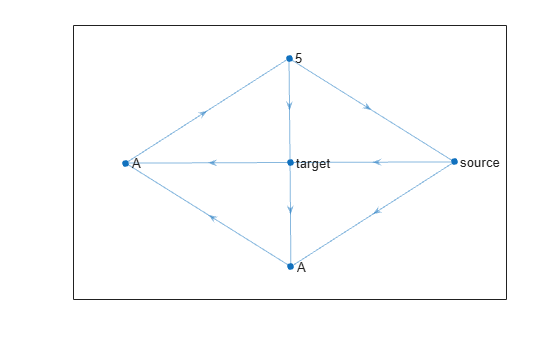# labelnode

Label graph nodes

## Syntax

``labelnode(H,nodeIDs,Labels)``

## Description

example

````labelnode(H,nodeIDs,Labels)` labels the nodes specified by `nodeIDs` with the character vectors or strings contained in `Labels`.```

## Examples

collapse all

Create and plot a graph.

```s = [1 1 2 2 3 4 5 5]; t = [2 3 3 4 4 5 1 2]; G = digraph(s,t); h = plot(G);```The `plot` function automatically labels the graph nodes with their node indices (or with their node names, if available). Use text labels for some of the graph nodes instead.

`labelnode(h,[1 2],{'source' 'target'})`Label node 3 and node 4 as `'A'`. Since the node labels do not change the underlying graph, `G`, the labels can contain duplicate names (the official node names in `G` must always be unique).

`labelnode(h,[3 4],'A')`## Input Arguments

collapse all

Input graph plot, specified as a `GraphPlot` object. Use the `graph` or `digraph` functions to create a graph, and then use `plot` with an output argument to return a `GraphPlot` object.

Example: `H = plot(G)`

Node identifiers, specified as a logical vector or as one or more node indices or node names. If `nodeIDs` is a logical vector, then its length must match the number of nodes in the graph.

This table shows the different ways to refer to one or more nodes either by their numeric node indices or by their node names.

FormSingle NodeMultiple Nodes
Node index

Scalar

Example: `1`

Vector

Example: `[1 2 3]`

Node name

Character vector

Example: `'A'`

Cell array of character vectors

Example: `{'A' 'B' 'C'}`

String scalar

Example: `"A"`

String array

Example: `["A" "B" "C"]`

Node labels, specified as a character vector or a cell array of character vectors.

• If `Labels` is a character vector or string scalar, then `labelnode` uses that label for each node specified by `nodeIDs`.

• If `Labels` is a cell array or string array, then it must contain a label for each node specified by `nodeIDs`.

Data Types: `char` | `cell` | `string`

### Topics

Introduced in R2015b